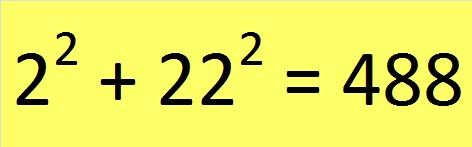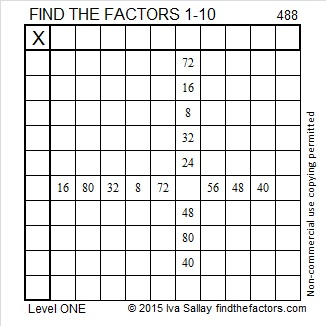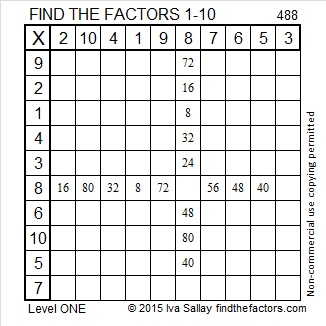# 488 and Level 1

I learned about a fun addition fact from OEIS.org. Look at all the 2’s in the sum and also a couple of powers of 2 in the hundred’s, ten’s, and one’s places of 488.Since 488 is the sum of two squares, it is also the hypotenuse of a Pythagorean triple: 88-480-488. The greatest common factor of those three numbers should be easy to spot.

488 is also divisible by 4 so its square root can be reduced. 488 ÷ 4 = 122. We can divide 122 by 2 to get 61 to verify that 122 has no square factors, but since it doesn’t we get this one layer cake:We simplify the square root by taking the square root of everything on the outside of the cake: √488 = (√4)(√122) = 2√122.Print the puzzles or type the solution on this excel file: 10 Factors 2015-05-11

—————————————————————————————————

• 488 is a composite number.
• Prime factorization: 488 = 2 x 2 x 2 x 61, which can be written 488 = (2^3) x 61
• The exponents in the prime factorization are 3 and 1. Adding one to each and multiplying we get (3 + 1)(1 + 1) = 4 x 2 = 8. Therefore 488 has exactly 8 factors.
• Factors of 488: 1, 2, 4, 8, 61, 122, 244, 488
• Factor pairs: 488 = 1 x 488, 2 x 244, 4 x 122, or 8 x 61
• Taking the factor pair with the largest square number factor, we get √488 = (√4)(√122) = 2√122 ≈ 22.09072—————————————————————————————————This site uses Akismet to reduce spam. Learn how your comment data is processed.